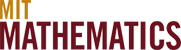Geometry

The modern discipline of geometry is affecting virtually every branch of mathematics, and is in a period of great progress. Many old problems are being solved using techniques from the area (notably Perelman's resolution of the Poincaré conjecture) and new directions are being pioneered. An important theme in this area has been the development and use of sophisticated techniques from the theory of PDEs to study natural equations that arise in geometry. The Atiyah-Singer index theorem from the 1960s connects the theory of linear PDEs to topology and geometry. The development of tools for nonlinear PDEs in geometry has been slower but has led to many of the most dramatic developments in mathematics including Donaldson's breakthroughs in the theory of four-manifolds using the Yang-Mills equations of high-energy physics.

The study of lower dimensional manifolds (dimensions four or less) has particular significance to theoretical physics and has many applications. Floer homology is a mathematically rigorous way of constructing parts of a quantum field theory. Another important and growing area is the mathematics of general relativity. The Lorentz version of the Einstein equations is now at the cutting edge of our hyperbolic PDE technology. One branch of geometric analysis involves the recovery of a function from its integrals over various domains. A well-known application of this idea is Computed Tomography Scanning (CT scans).

The research interest of this group covers geometric analysis as well as symplectic topology and its role in mirror symmetry, low dimensional topology and gauge theory, Riemannian geometry and minimal surfaces and mathematical physics.

Faculty

Tobias Colding

Tristan Collins

Victor Guillemin

Larry Guth

William Minicozzi

Tom Mrowka

Paul Seidel